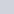# Vertical analysis

• This topic has 5 replies, 2 voices, and was last updated 1 month, 2 weeks ago byLynn Roden.
• Creator
Topic
• #232225Amir Badawy
Participant

How I can use vertical analysis  on Cash flows ?

Please by practical example

Viewing 5 replies - 1 through 5 (of 5 total)
• Author
Replies
• #232245Lynn Roden
HOCK international

Hello Amir Badawy,

I do not think a vertical analysis of a Statement of Cash Flows would be meaningful. A vertical common-size financial statement covers one year’s operating results and expresses each component as a percentage of a total. A Statement of Cash Flows presents net cash provided (an amount which may be either positive or negative) by operating, investing, and financing activities and also the net increase (or decrease) in cash, cash equivalents, and restricted cash that is the sum of the three activities. Any vertical analysis would be done by simply dividing each activity’s net cash flow by the total net increase or decrease in cash.

However, if some of those amounts are negative and some are positive, the result of calculating the percentages would not be meaningful. Dividing a negative number by a positive number or dividing a positive number by a negative number results in a percentage that does not have meaning. All the amounts must be positive or all must be negative for the resulting percentages to be meaningful.

Therefore, vertical analysis can be done on a Statement of Cash Flows only if all the numbers have the same sign. And since it cannot be done consistently, it is not useful.

Lynn

#232396Amir Badawy
Participant

Thanks Lynn

But we can use common size cash flow as a percentage from revenue

Doesn’t it ?

#232400Lynn Roden
HOCK international

Hello Amir Badawy,

I just realized why you have asked this question. I noticed the explanation in the HOCK CMA Part 2, Vol. 1 textbook for vertical common-size financial statements that says:

“Line items on the income statement and statement of cash flows are presented as a percentage of sales revenue.”

I do not know why “statement of cash flows” is included in that, because it does not belong there. Vertical analysis is done on either the balance sheet or the income statement. The percentages are always percentages of one line item on a statement divided by another line item on the same statement. The statement of cash flows is a separate statement from the income statement, where revenue is reported.

So “statement of cash flows” needs to be deleted from that sentence, and I will see that that is done in the next update to that book.

I am very sorry for the confusing information. Thank you for persisting!

Lynn

#232453Amir Badawy
Participant

Thanks Lynn

I think We can used vertical analysis on analysis Cash flow statement divided by revenue, I researched about this issue and I found we can use this for forecast cash flows as per attached

My source Schweser CFA level 1

#232456Lynn Roden
HOCK international

Hello Amir Badawy,

Thank you for sharing that. I have researched it a little more, and I did find several other references to using a statement of cash flows where the operating activities section has been prepared according to the indirect method to do vertical analysis by expressing each line item on the cash flow statement as a percentage of revenue.

I can see the usefulness of doing vertical analysis with a statement of cash flows where the operating activities section has been prepared according to the direct method. As your attachment suggests, on such a statement, each inflow of cash can be expressed as a percentage of total cash inflows, and each outflow of cash can be expressed as a percentage of total cash outflows. When vertical analysis is done that way, you are dividing positive numbers by positive numbers and negative numbers by negative numbers, and the percentages that result have meaning. But doing that is not possible when the operating activities section of the cash flow statement has been prepared according to the indirect method because the SCF does not present total cash inflows from operating activities separately from total cash outflows from operating activities.

I still think that dividing a negative number by a positive number or vice versa results in a percentage that is meaningless. Therefore, I do not see the usefulness of doing vertical analysis with a statement of cash flows where the operating activities section has been prepared according to the indirect method and the line items are expressed as percentages of revenue.

Apparently the ICMA feels that vertical analysis on a statement of cash flows is not necessary, because their Learning Outcome Statement says this:

“for the balance sheet and income statement, prepare and analyze common-size financial statements; i.e., calculate percentage of assets and sales, respectively; also called vertical analysis”

Therefore, I do not think you need to worry about vertical analysis of a cash flow statement for the CMA exams.

Lynn

• This reply was modified 1 month, 2 weeks ago byLynn Roden.
Viewing 5 replies - 1 through 5 (of 5 total)
• You must be logged in to reply to this topic.